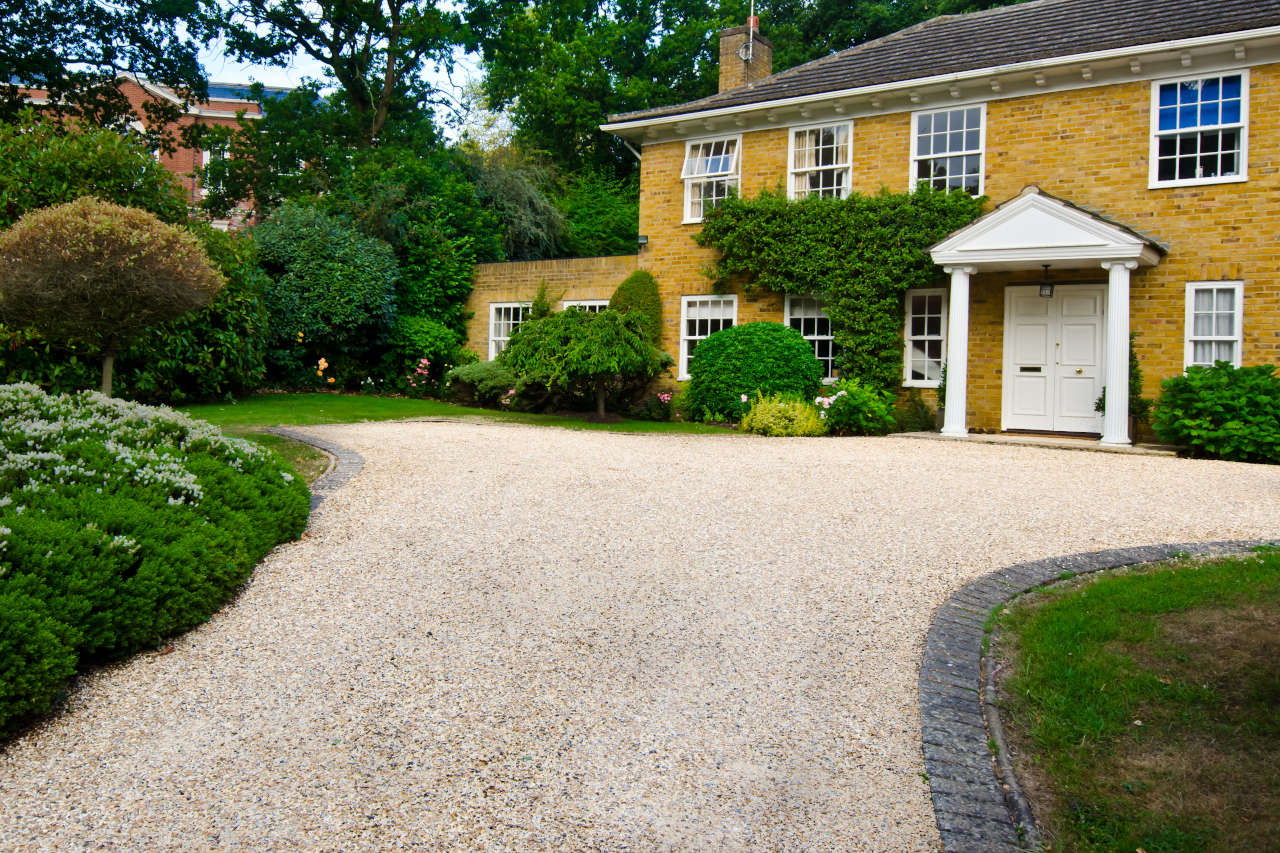# Gravel Driveway Calculator – Estimate Material for a Gravel Driveway

Find the amount of gravel needed for a driveway by entering the dimensions of the driveway below. Add the price per cubic yard to estimate the cost of the stone.

Optionally enter the price per cubic yard
\$

## Crushed Stone Material Estimate:

cubic yards

tons*
*Estimate only - weight varies by material type.
Estimated Material Cost

material cost
Learn how we calculated this below

## How to Estimate a Gravel DrivewayCrushed stone is a common material to use for a driveway because it offers a nice clean look, is resistant to weeds, is affordable, is easy to maintain, and is easy to install. Gravel is commonly sold by the cubic yard, so to find the material needed for a driveway, you need to find the volume in yards.

### Calculate How Many Cubic Yards of Gravel You Need

Estimating gravel driveway materials is as simple as estimating the cubic yards of volume the driveway needs to be. Cubic yards are found by multiplying the length by the width by the depth in yards.

Find the driveway’s length and width in yards by dividing the measurement in feet by 3. A 100’ measuring tape or measuring wheel may be needed to find these measurements depending on how long the driveway is.

Divide the measurement in inches by 36 to find the driveway’s depth in yards. Most driveways should have a depth of 6-8″ of gravel.

The formula to find volume is length × width × depth. Multiply the width by the length by the depth in yards to find the cubic yards of gravel needed.

### Calculate How Many Tons of Gravel are Needed

Gravel is sometimes sold by the ton instead of the cubic yard. If you know how many cubic yards of gravel are needed then find the number of tons needed by multiplying the cubic yards by 1.35. Our gravel calculator simplifies the process of estimating material in tons.

For example, see how to calculate that 50 cubic yards of gravel weighs 67.5 tons with this formula.

50 yds3× 1.35 = 67.5

Note that different materials have different weights. Crushed gravel weights about 1.35 tons per cubic yard, but other materials may vary in weight.

Gravel driveways can range in price depending on their size and the type of gravel used, learn more about what impacts the cost.

See our concrete driveway calculator if you want to compare to using concrete for your project, or check out our stone calculator.